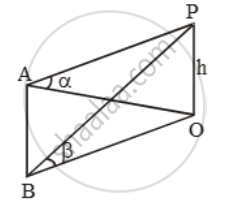Share

# The elevation of a tower at a station A due north of it is α and at a station B due west of A is β. Prove that the height of the tower is - CBSE Class 10 - Mathematics

#### Question

The elevation of a tower at a station A due north of it is α and at a station B due west of A is β. Prove that the height of the tower is \frac{AB\sin \alpha \sin \beta }{\sqrt{\sin^{2}\alpha -\sin ^{2}\beta

#### Solution

Let OP be the tower and let A be a point due north of the tower OP and let B be the point due west of A. Such that ∠OAP = and ∠OBP = Let h be the height of the tower.

In right angled triangles OAP and OBP, we have\tan \alpha =\frac{h}{OA}\text{ and }\tan \beta =\frac{h}{OB}

⇒ OA = h cot α and OB = h cot β.

In ∆OAB, we have

OB^2 = OA^2 + AB^2

⇒ AB^2 = OB^2 – OA^2

⇒ AB^2 = h^2 cot^2 β– h2 cot^2 α

⇒ AB^2 = h^2 [cot^2 β– cot^2 α]

⇒ AB^2 = h^2 [(cosec^2 β– 1) – (cosec^2 α– 1)]

⇒ AB^2 = h^2 (cosec^2 β– cosec^2 α)

\Rightarrow AB^{2}=h^{2}( \frac{\sin ^{2}\alpha -\sin^{2}\beta }{\sin ^{2}\alpha \sin ^{2}\beta ))

\Rightarrow h=\frac{AB\sin \alpha \sin \beta }{\sqrt{\sin ^{2}\alpha-\sin ^{2}\beta

Is there an error in this question or solution?

#### Video TutorialsVIEW ALL 

Solution The elevation of a tower at a station A due north of it is α and at a station B due west of A is β. Prove that the height of the tower is Concept: Heights and Distances.
S